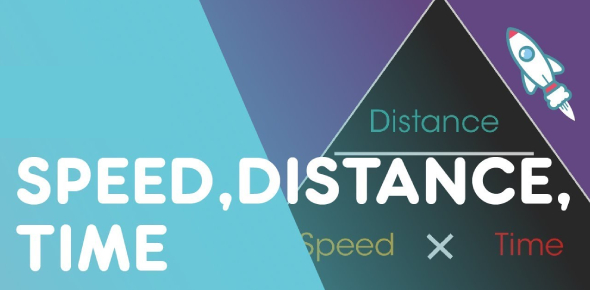# Speed Distance And Time Quiz

10 Questions | Attempts: 6558
ShareSettingsSpeed, Distance, and Time are fundamentals of physics. This is a quiz to test students' ability to analyze and solve conceptual problems. The quiz contains various numerical and statement-based questions that will test your understanding of the topic. It has a wide coverage of easy, medium, to hard-level questions. So let's see how prepared you are to attempt these questions. If you find the quiz helpful, do share it with your friends. All the best!

• 1.
How fast is a runner travelling if she covers 100m in 12s?
• A.

0.12m/s

• B.

1200m/s

• C.

24m/s

• D.

8.33m/s

• E.

6.23m/s

• 2.
A cyclist travels 125km in 5hours. How fast is the cyclist moving?
• A.

25 mph

• B.

25km/h

• C.

0.04mph

• D.

0.04km/h

• E.

625km/h

• 3.
A plane travels at 600km/h. How far will it travel in 3hr 30min?
• A.

171km

• B.

181km

• C.

1980km

• D.

2100km

• E.

3200km

• 4.
A snail is crawling for 45s at a speed of 0.35cm/s. How far does it travel?
• A.

1.286m

• B.

128.6cm

• C.

15.75cm

• D.

45.35cm

• E.

1.575m

• 5.
The journey from Manster to Lendemon is 186km. If the train travels at 140km/h, how long will journey take?
• A.

13.2hrs

• B.

0.75hrs

• C.

0.96hrs

• D.

1.22hrs

• E.

1.32hrs

• 6.
A sprinter runs the 200m at 8.6m/s. How long does she take to complete the race?
• A.

23.3s

• B.

23.3min

• C.

23.3hrs

• D.

0.043s

• E.

1720s

• 7.
A Straight Sloping Line on a distance time graph means:-
• A.

Speeding Up

• B.

Slowing Down

• C.

Going Up hill

• D.

Going Down Hill

• E.

Constant Speed

• 8.
The gradient on a distance time graph represents:-
• A.

Energy

• B.

Force

• C.

Speed

• D.

Acceleration

• E.

Momentum

• 9.
A curved line which is getting steeper on a Distance-Time graph represents:-
• A.

Deceleration

• B.

Acceleration

• C.

Constant Speed

• D.

A Stationary Object

• E.

Kinetic Energy

• 10.
Speed and Velocity are different. Velocity means:-
• A.

Speed after a certain time

• B.

Speed in a particular direction

• C.

Speed with a particular force

• D.

Speed with a particular energy

• E.

Speed after a certain distance

## Related TopicsBack to top
×

Wait!
Here's an interesting quiz for you.